# Electrical Engineering - Circuit Theorems in AC Analysis

Exercise : Circuit Theorems in AC Analysis - General Questions
1.
Determine VTH when R1 is 180and XL is 90.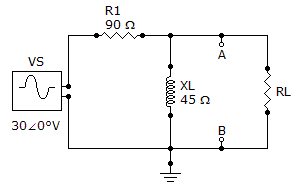135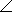63.4° V
13.563.4° V
12.20° V
1220° V
Explanation:
No answer description is available. Let's discuss.

2.
For the given circuit, find VTH for the circuit external to RL.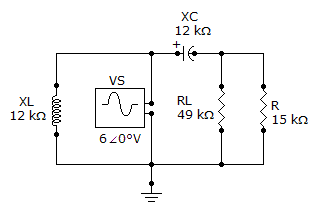4.6951.3° V
4.6938.7° V
46938.7° mV
60° V
Explanation:
No answer description is available. Let's discuss.

3.
Referring to the given circuit, find ZTH if R is 15 kand RL is 38 k.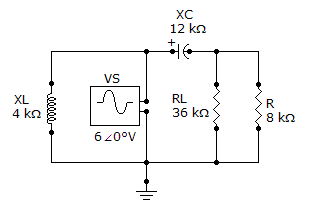89.82–51.3° k19.2–38.3° k9.38–51.3° k180–38.3° kExplanation:
No answer description is available. Let's discuss.

4.
For the circuit shown, determine ZTH for the portion of the circuit external to RL.66.7–33.7° k6.67–333.7° k14.4–56.3° k1.44–33.7° kExplanation:
No answer description is available. Let's discuss.

5.
Referring to the given circuit, what is ZTH if R1 is changed to 220?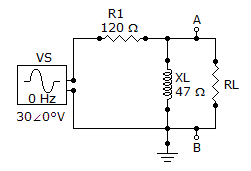22512.1°22577.9°4677.9°4612.1°